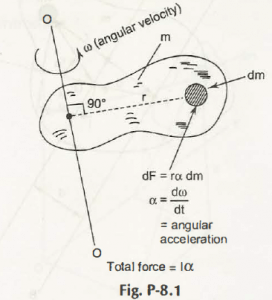Let the mass moment of inertia of differential mass about 0 – 0 axis be dm as shown in Fig. P-8.1.If it is perpendicular to plane of rotation of centre of mass, then it can be written as,

dI = r2 dm

or,

I = 2 dm

Where,

I = Mass Moment of Inertia

Keeping the mass density, p as constant, then we get-

dm = p dV

where,

dV = Element of Volume

Hence, from Fig. P-8.1,

I = 2p dV

= P2 dV

Links of Next Mechanical Engineering Topics:-### Customer Reviews

My Homework Help
Rated 5.0 out of 5 based on 510 customer reviews at